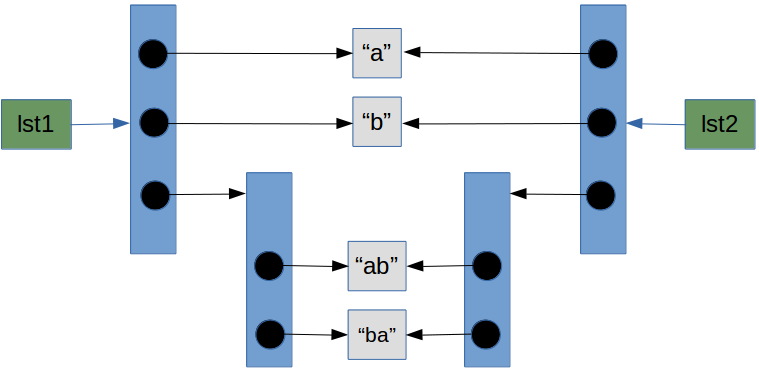## Shallow and Deep Copy

### IntroductionIn this chapter, we will cover the question of how to copy lists and nested lists. Trying to copy lists can be a stumping experience for newbies. But before we would like to summarize some insights from the previous chapter "Data Types and Variables". Python even shows a strange behaviour for beginners of the language - in comparison with some other traditional programming languages - when assigning and copying simple data types like integers and strings. The difference between shallow and deep copying is only relevant for compound objects, i.e. objects containing other objects, like lists or class instances.

In the following code snippet, y points to the same memory location as X. We can see this by applying the id() function on x and y. However, unlike "real" pointers like those in C and C++, things change, when we assign a new value to y. In this case y will receive a separate memory location, as we have seen in the chapter "Data Types and Variables" and can see in the following example:

x = 3
y = x
print(id(x), id(y))

140709828469200 140709828469200

y = 4
print(id(x), id(y))

140709828469200 140709828469232

print(x,y)

3 4


But even if this internal behaviour appears strange compared to programming languages like C, C++ and Perl, yet the observable results of the assignments answer our expectations. However, it can be problematic, if we copy mutable objects like lists and dictionaries.

Python creates only real copies, if it has to, i.e. if the user, the programmer, explicitly demands it.

We will introduce you the most crucial problems, which can occur when copying mutable objects, i.e. when copying lists and dictionaries.

### Copying a list

colours1 = ["red", "blue"]
colours2 = colours1
print(colours1)

['red', 'blue']

print(colours2)

['red', 'blue']

print(id(colours1),id(colours2))

1263006702920 1263006702920

colours2 = ["rouge", "vert"]
print(colours1)

['red', 'blue']

print(colours2)

['rouge', 'vert']

print(id(colours1),id(colours2))

1263006702920 1263006702984


In the example above a simple list is assigned to colours1. This list is a so-called "shallow list", because it doesn't have a nested structure, i.e. no sublists are contained in the list. In the next step we assign colours1 to colours2.

The id() function shows us that both variables point at the same list object, i.e. they share this object.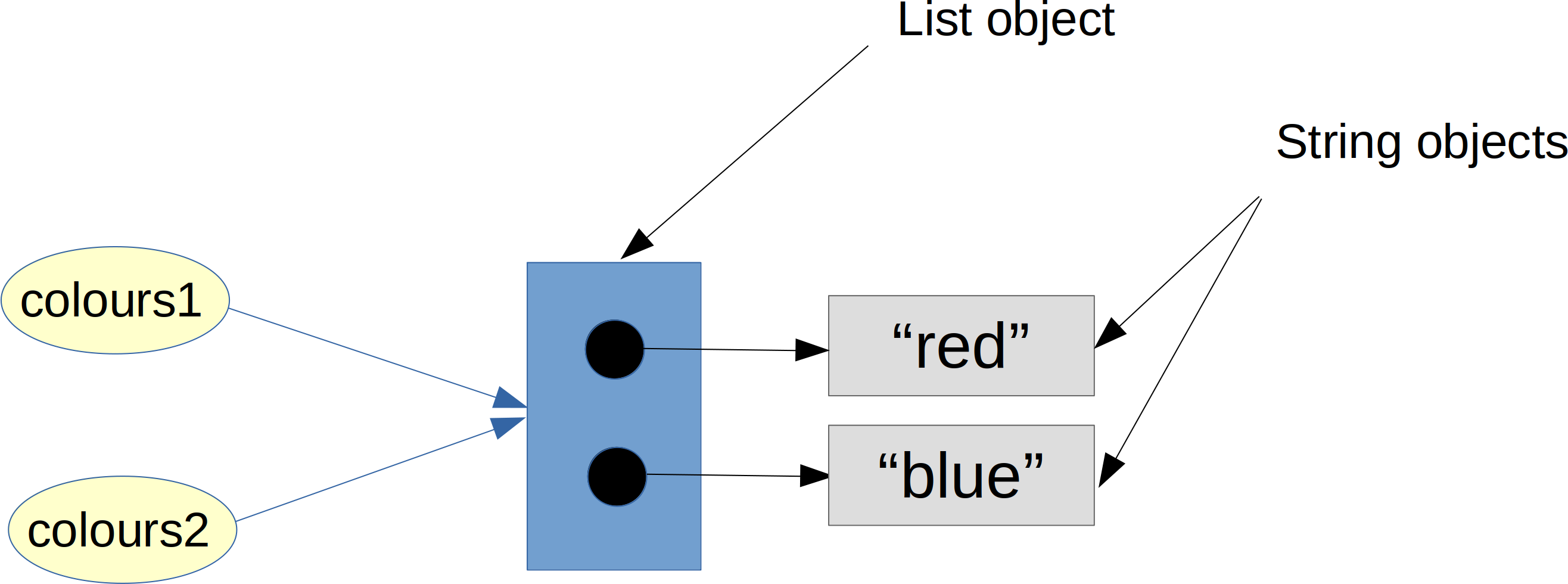We have two variable names "colours1" and "colours2", which we have depicted as green boxes. The blue box symbolizes the list object. A list object consists of references to other objects. In our example the list object, which is references by both variables, references two string objects, i.e. "red" and "blue". Now we have to examine, what will happen, if we change just one element of the list of colours2 or colours1.

Now we want to see, what happens, if we assign a new list object to colours2. As expected, the values of colours1 remained unchanged. Like it was in our example in the chapter "Data Types and Variables", a new memory location had been allocated for colours2, as we have assigned a completely new list, i.e. a new list object to this variable.

colours1 = ["red", "blue"]
colours2 = colours1
print(id(colours1),id(colours2))

1263006718088 1263006718088

colours2 = "green"
print(id(colours1),id(colours2))

1263006718088 1263006718088

print(colours1)

['red', 'green']

print(colours2)

['red', 'green']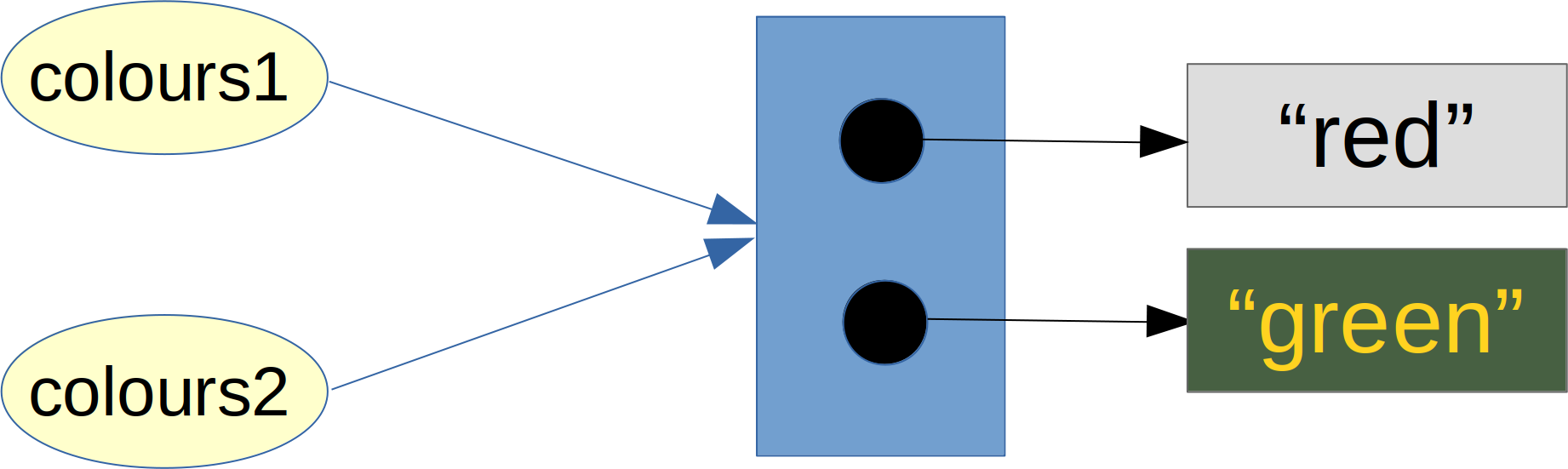Let's see, what has happened in detail in the previous code. We assigned a new value to the second element of colours2, i.e. the element with the index 1. Lots of beginners will be surprised as the list of colours1 has been "automatically" changed as well. Of course, we don't have two lists: We have only two names for the same list!

The explanation is that we didn't assign a new object to colours2. We changed colours2 inside or as it is usually called "in-place". Both variables "colours1" and "colours2" still point to the same list object.

### Copy with the Slice Operator

It's possible to completely copy shallow list structures with the slice operator without having any of the side effects, which we have described above:

list1 = ['a','b','c','d']
list2 = list1[:]
list2 = 'x'
print(list2)

['a', 'x', 'c', 'd']

print(list1)

['a', 'b', 'c', 'd']


But as soon as a list contains sublists, we have another difficulty: The sublists are not copied but only the referenced to the sublists. The following example list "lst2" contains one sublist. We create a shallow copy with the slicing operator.

lst1 = ['a','b',['ab','ba']]
lst2 = lst1[:]


The following diagram depicts the data structure after the copying. We can see that both lst1 and lst2 point to the same object, i.e. the sublist: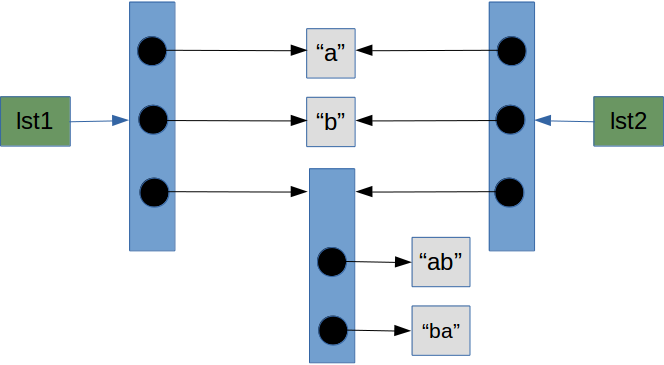If you assign a new value to the 0th or the 1st index of one of the two lists, there will be no side effect.

lst1 = ['a','b',['ab','ba']]
lst2 = lst1[:]
lst2 = 'c'
print(lst1)

['a', 'b', ['ab', 'ba']]

print(lst2)

['c', 'b', ['ab', 'ba']]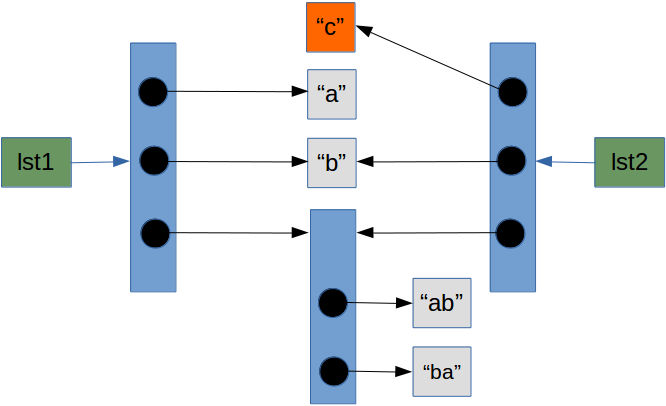Problems arise, if you change one of the elements of the sublist:

lst2 = 'd'
print(lst1)

['a', 'b', ['ab', 'd']]

print(lst2)

['c', 'b', ['ab', 'd']]


The following diagram depicts the situation after we have executed the code above. We can see that both lst1 and lst2 are affected by the assignment lst2 = 'd':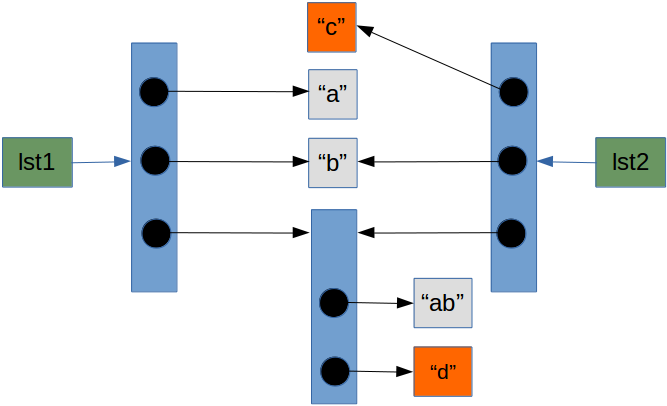### Using the Method Deepcopy from the Module copy

A solution to the described problems are provided by the module "copy". This module provides the method "deepcopy", which allows a complete or deep copy of an arbitrary list, i.e. shallow and other lists.

Let's use deepcopy for our previous list:

from copy import deepcopy
lst1 = ['a','b',['ab','ba']]
lst2 = deepcopy(lst1)
lst1

Output::
['a', 'b', ['ab', 'ba']]
lst2

Output::
['c', 'b', ['ab', 'd']]
id(lst1)

Output::
1263005552200
id(lst2)

Output::
1263006533448
id(lst1)

Output::
1262934748208
id(lst2)

Output::
1262934630704
id(lst2)

Output::
1263005550280
id(lst1)

Output::
1263005550280

We can see by using the id function that the sublist has been copied, because id(lst2) is different from id(lst1). An interesting fact is that the strings are not copied: lst1 and lst2 reference the same string. This is true for lst1 and lst2 as well, of course. The following diagram shows the situation after copying the list: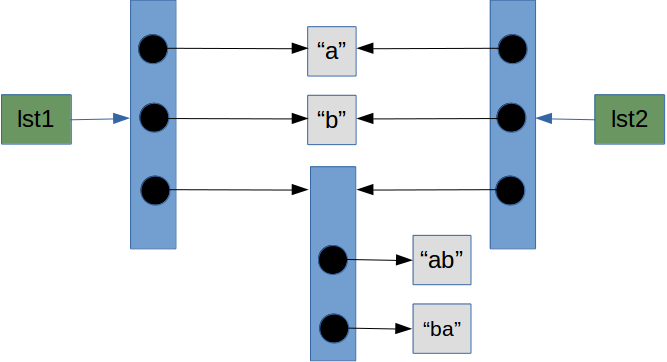lst2 = "d"
lst2 = "c"
print(lst1)

['a', 'b', ['ab', 'd']]

print(lst2)

['c', 'b', ['ab', 'd']]


Now the data structure looks like this: